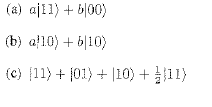Quantum Mechanics Entangled States

Homework Statement

I've been asked to find out ifare entangled states or not

Homework Equations

I thought an entangled state was one where a measurement of one qubit revealed the nature of the other qubit in the state

The Attempt at a Solution

If I am correct in my definition of an entangled state then a) and b) are entangled as measuring a 1 or a zero in a) reveals the state of the 2nd qubit, and there is only one type of qubit in b). c isn't entangled as measurement of q1 does not reveal the nature of q2?
Could someone please tell me if I am correct or not, and if not why not? Many thanks!

$$\vert\psi_{AB}\rangle=a\vert 00\rangle+b\vert 10\rangle+c\vert 01\rangle+d\vert 11\rangle$$
then this state is entangled if and only if it cannot be written as $\vert\psi_{A}\rangle\otimes \vert\psi_{B}\rangle$